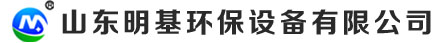15963635951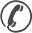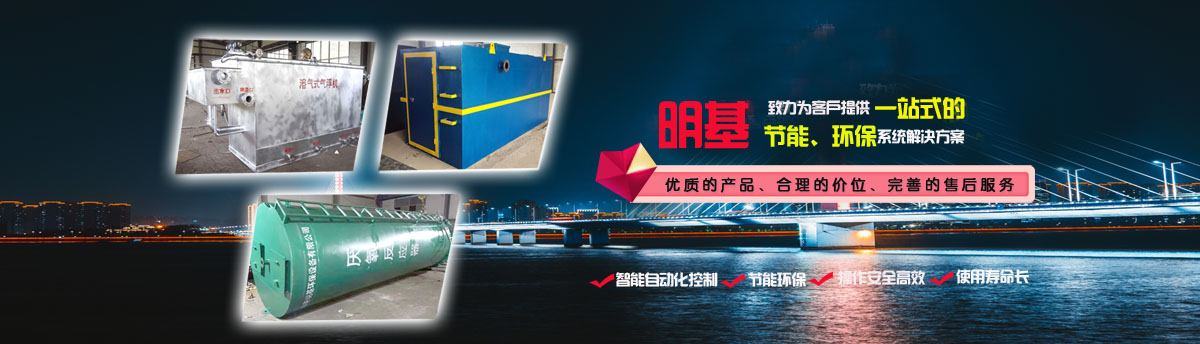15963635951

1、进水水质情况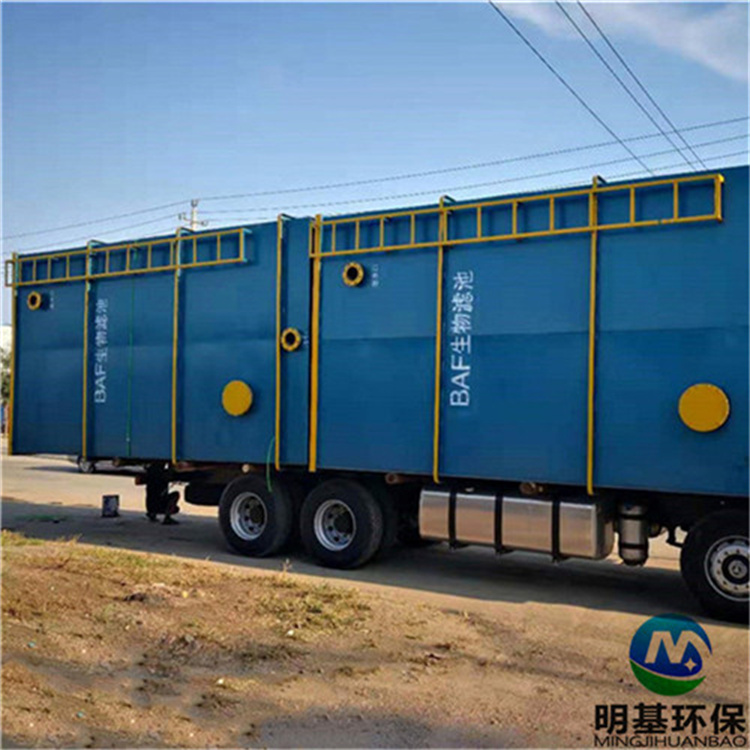Q=12000m³/d
COD≤60mg/L
BOD5≤30mg/L

2、采用硝化、反硝化生物脱氮工艺时，技术要求

1. 反硝化生物滤池(DN池)计算
（1）按反硝化容积负荷法计算
A=W/H0
W=Q*CN/(1000*qTN)

W--滤料总体积（m³）
H0---滤料装填高度（m）
CN--反硝化滤池进、出水硝酸盐氮浓度差值（mg/L）
Q—设计污水流量（m³/d）
qTN反硝化容积负荷（KgNO3--N）/m³.d
• 进水硝酸氮浓度取大值：50mg/L,出水取小5mg/L,则CN为45mg/L
• 反硝化容积负荷qTN=0.8 KgNO3--N/（m³.d），规范取值范围为（0.8 -1.2）KgNO3--N/（m³.d）
• 滤料总体积： W=Q*CN/(1000*qTN)=12000*45/（1000*0.8）=675m³
• 滤料装填高度：H0=3.5m规范取值范围为（2.5m-4.5m）
• 滤池总面积：A=W/H0=675/3.5=193㎡
• 滤池数量n=2座
• 单池面积：W0=A/2=193/2=96.5㎡（单池面积＜100㎡），符合规范要求。
（2）按空床停留时间计算
A=QT/24q
q= H0/t

QT –设计污水量与消化液回流量之和（m³/d）含
H0---滤料装填高度（m）
t空床水力停留时间（h），取值范围20-30min
q—滤池水力表面负荷（m³/㎡.h），即滤速（取值范围8.0-10），含回流
①混合液回流比R=μ/1-μ,取值范围100%-400%，取200%
②q—滤池水力表面负荷，取8.0m³/（㎡.h），符合要求
③滤池总面积A=QT/24q =12000*3/（24*8）=187.5㎡
④空床停留时间t= H0/q=0.375h=22.5min（符合要求）
（3）反硝化生物滤池确定
①反硝化生物滤池面积确定

②滤池高度H= H0+h0+h1+h2+h3+h4
H0---滤料装填高度（m）（取值范围2.5-4.0m）
h0---承托层高度（m）（取值范围0.3-0.5m）
h1---缓冲配水区高度（m）（取值范围1.35-1.5m）
h2---清水区高度（m）（取值范围1.0-1.5m）
h3---滤板厚度高（m）

③反硝化生物滤池确定

（4）反硝化生物滤池反冲洗设计
①　空气冲洗强度：12-16L/㎡.S
设计采用12L/㎡.S，单池冲洗空气量Q=12*100=1200 L/S=72m³/min
②　水冲洗强度4-6L/㎡.S
设计采用4L/㎡.S，单池冲洗水量Q=4*100=400L/S=1440m³/h
（5）长柄滤头设计

（6）外加碳源设计

1. 硝化曝气生物滤池（N池）计算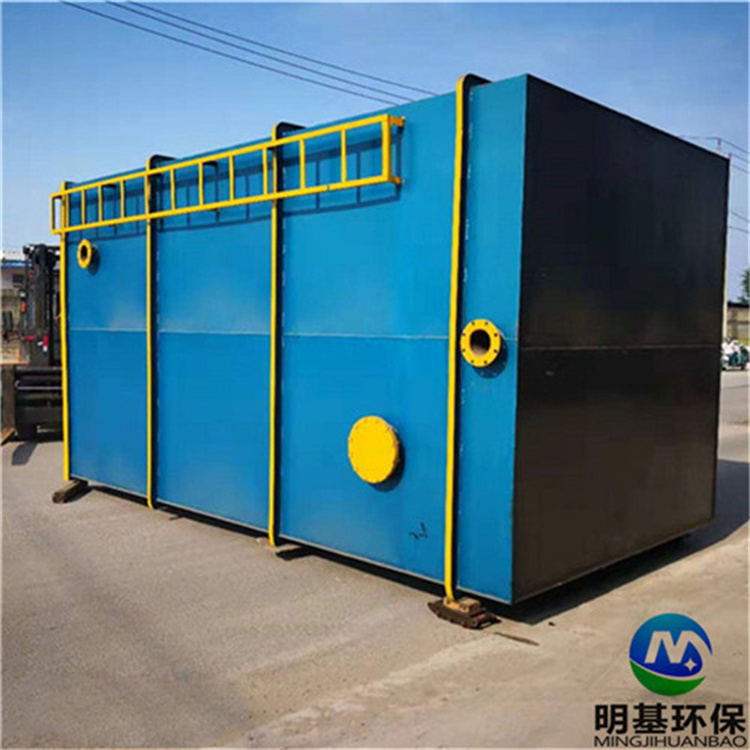（1）按硝化容积负荷法计算
A=W/H0
W=Q*CTKN/(1000*qNH3-N)

W--滤料总体积（m³）
H0--滤料装填高度（m）
CTKN --进、出硝化滤池凯式氮浓度差值（mg/L）
Q—设计污水流量（m³/d）
qNH3-N硝化容积负荷（KgNH3-N）/m³.d
• 进水总凯式氮浓度40mg/L,出水设计8mg/L,则CTKN为32mg/L
• 硝化容积负荷qNH3-N =0.6 KgNH3-N/（m³.d），规范取值范围为（0.6 -1.0）KgNH3-N/（m³.d）
• 滤料总体积W=Q*CTKN /(1000*qNH3-N)=12000*32/（1000*0.6）=640m³
• 滤料装填高度：H0=3.5m，规范取值范围为（2.5m-4.5m）
• 滤池总面积：A=W/H0=640/3.5=182㎡
• 滤池数量n=2座
• 单池面积：W0=A/2=182/2=91㎡（单池面积＜100㎡），符合规范要求。
（2）按空床停留时间计算
A=Q/24q
q= H0/t

Q –设计污水量（m³/d）
H0---滤料装填高度（m）
t空床水力停留时间（h），取值范围30-45min
q—滤池水力表面负荷（m³/㎡.h），即滤速（取值范围3-12）
①空床停留时间t取40min=0.67h（符合要求）
②滤池水力表面负荷q= H0/t=3.5/0.67=5.2 m³/(㎡.h）.符合要求
③滤池总面积A=Q/24q =12000/（24*5.2）=96㎡
④空床停留时间t= H0/q=0.375h=22.5min（符合要求）
（3）反硝化曝气生物滤池确定
①反硝化生物滤池面积确定

②滤池高度H= H0+h0+h1+h2+h3+h4
H0---滤料装填高度（m）（取值范围2.5-4.0m）
h0---承托层高度（m）（取值范围0.3-0.5m）
h1---缓冲配水区高度（m）（取值范围1.35-1.5m）
h2---清水区高度（m）（取值范围1.0-1.5m）
h3---滤板厚度高（m）

③反硝化曝气生物滤池确定

（4）硝化曝气生物滤池反冲洗设计
• 空气冲洗强度：12-16L/㎡.S
设计采用12L/㎡.S，单池冲洗空气量Q=12*95=1140 L/S=68.4m³/min，风压：58.8Kpa
• 水冲洗强度4-6L/㎡.S
设计采用4L/㎡.S，单池冲洗水量Q=4*95=380L/S=1368m³/h
（5）长柄滤头设计

（6）曝气系统设计

1、进水水质情况
Q=12000m³/d
COD≤60mg/L
BOD5≤30mg/L

2、采用硝化、反硝化生物脱氮工艺时，技术要求

1. 反硝化生物滤池(DN池)计算
（1）按反硝化容积负荷法计算
A=W/H0
W=Q*CN/(1000*qTN)

W--滤料总体积（m³）
H0---滤料装填高度（m）
CN--反硝化滤池进、出水硝酸盐氮浓度差值（mg/L）
Q—设计污水流量（m³/d）
qTN反硝化容积负荷（KgNO3--N）/m³.d
• 进水硝酸氮浓度取大值：50mg/L,出水取小5mg/L,则CN为45mg/L
• 反硝化容积负荷qTN=0.8 KgNO3--N/（m³.d），规范取值范围为（0.8 -1.2）KgNO3--N/（m³.d）
• 滤料总体积： W=Q*CN/(1000*qTN)=12000*45/（1000*0.8）=675m³
• 滤料装填高度：H0=3.5m规范取值范围为（2.5m-4.5m）
• 滤池总面积：A=W/H0=675/3.5=193㎡
• 滤池数量n=2座
• 单池面积：W0=A/2=193/2=96.5㎡（单池面积＜100㎡），符合规范要求。
（2）按空床停留时间计算
A=QT/24q
q= H0/t

QT –设计污水量与消化液回流量之和（m³/d）含
H0---滤料装填高度（m）
t空床水力停留时间（h），取值范围20-30min
q—滤池水力表面负荷（m³/㎡.h），即滤速（取值范围8.0-10），含回流
①混合液回流比R=μ/1-μ,取值范围100%-400%，取200%
②q—滤池水力表面负荷，取8.0m³/（㎡.h），符合要求
③滤池总面积A=QT/24q =12000*3/（24*8）=187.5㎡
④空床停留时间t= H0/q=0.375h=22.5min（符合要求）
（3）反硝化生物滤池确定
①反硝化生物滤池面积确定

②滤池高度H= H0+h0+h1+h2+h3+h4
H0---滤料装填高度（m）（取值范围2.5-4.0m）
h0---承托层高度（m）（取值范围0.3-0.5m）
h1---缓冲配水区高度（m）（取值范围1.35-1.5m）
h2---清水区高度（m）（取值范围1.0-1.5m）
h3---滤板厚度高（m）

③反硝化生物滤池确定

（4）反硝化生物滤池反冲洗设计
①　空气冲洗强度：12-16L/㎡.S
设计采用12L/㎡.S，单池冲洗空气量Q=12*100=1200 L/S=72m³/min
②　水冲洗强度4-6L/㎡.S
设计采用4L/㎡.S，单池冲洗水量Q=4*100=400L/S=1440m³/h
（5）长柄滤头设计

（6）外加碳源设计